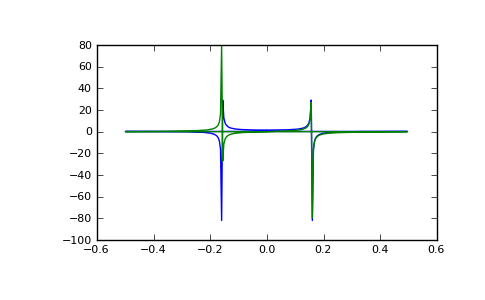# numpy.fft.fft¶

numpy.fft.fft(a, n=None, axis=-1)

Compute the one-dimensional discrete Fourier Transform.

This function computes the one-dimensional n-point discrete Fourier Transform (DFT) with the efficient Fast Fourier Transform (FFT) algorithm [CT].

Parameters: a : array_like Input array, can be complex. n : int, optional Length of the transformed axis of the output. If n is smaller than the length of the input, the input is cropped. If it is larger, the input is padded with zeros. If n is not given, the length of the input (along the axis specified by axis) is used. axis : int, optional Axis over which to compute the FFT. If not given, the last axis is used. out : complex ndarray The truncated or zero-padded input, transformed along the axis indicated by axis, or the last one if axis is not specified. IndexError : if axes is larger than the last axis of a.

numpy.fft
for definition of the DFT and conventions used.
ifft
The inverse of fft.
fft2
The two-dimensional FFT.
fftn
The n-dimensional FFT.
rfftn
The n-dimensional FFT of real input.
fftfreq
Frequency bins for given FFT parameters.

Notes

FFT (Fast Fourier Transform) refers to a way the discrete Fourier Transform (DFT) can be calculated efficiently, by using symmetries in the calculated terms. The symmetry is highest when n is a power of 2, and the transform is therefore most efficient for these sizes.

The DFT is defined, with the conventions used in this implementation, in the documentation for the numpy.fft module.

References

 [CT] Cooley, James W., and John W. Tukey, 1965, “An algorithm for the machine calculation of complex Fourier series,” Math. Comput. 19: 297-301.

Examples

```>>> np.fft.fft(np.exp(2j * np.pi * np.arange(8) / 8))
array([ -3.44505240e-16 +1.14383329e-17j,
8.00000000e+00 -5.71092652e-15j,
2.33482938e-16 +1.22460635e-16j,
1.64863782e-15 +1.77635684e-15j,
9.95839695e-17 +2.33482938e-16j,
0.00000000e+00 +1.66837030e-15j,
1.14383329e-17 +1.22460635e-16j,
-1.64863782e-15 +1.77635684e-15j])
```
```>>> import matplotlib.pyplot as plt
>>> t = np.arange(256)
>>> sp = np.fft.fft(np.sin(t))
>>> freq = np.fft.fftfreq(t.shape[-1])
>>> plt.plot(freq, sp.real, freq, sp.imag)
>>> plt.show()
```

In this example, real input has an FFT which is Hermitian, i.e., symmetric in the real part and anti-symmetric in the imaginary part, as described in the numpy.fft documentation.

Output(png, pdf)

#### Previous topic

numpy.fft.ifftshift

numpy.fft.ifft